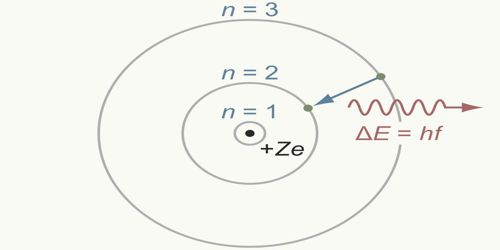# Bohr’s Atom Model

Bohr’s Atom Model

In 1913, famous Dutch scientist Niels Bohr proposed this model of an atom and in 1922 he got Nobel Prize for this discovery. Bohr proposed that the laws of classical mechanics and the electromagnetism break down in case of atoms. Actually, he applied quantum mechanics in Rutherford’s atom model and by a revolutionary expansion of quantum theory he explained the atomic spectra. In honor of his name, this model is called Bohr’s atom model. In this model, he tried to remove the defects of Rutherford’s atom model. He applied the following postulates in Rutherford’s atom model. These postulates are called Bohr’s postulates.

Bohr’s postulates

First postulate:

An electron, while orbiting in a permanent orbit its total angular momentum will be an integral multiple of h/2π, here ‘h’ is the Planck’s constant.

This means that an electron having mass ‘m’ orbiting with a velocity ‘v’ in a permanent orbit of radius r, its angular momentum, mvr = nh/2π, here, n is an integer. For different orbits the values of n are different. With respect to the position of the nucleus for the first, second and third orbits etc., n = 1, 2, 3 etc., respectively and can never be zero; n is called the principal quantum number.

Second postulate:

Electrons in an atom cannot revolve around the nucleus in all probable orbits; rather they rotate in certain fixed prescribed circular orbits. These orbits are called permanent and non-radiating orbits. When the electron revolves in a permanent orbit, it does not emit electromagnetic radiation as predicted by electromagnets theory of light and the trajectory of an electron does not approach the nucleus in a spiral way. Hence Bohr’s atom model overcomes the limitation of Rutherford’s atom model.

Third postulate:

Whenever an electron jumps from a convenient orbit to another convenient orbit, then radiation or absorption of energy takes place. If the transition is from an orbit of higher energy to an orbit of lower energy then radiation of energy takes place. On the other hand, if an electron jumps from a lower orbit to a higher Electron orbit, then it absorbs energy.